# 动手实践丨基于 ModelAtrs 使用 A2C 算法制作登月器着陆小游戏

• 2022-11-23
中国香港
• 本文字数：5758 字

阅读完需：约 19 分钟LunarLander 是一款控制类的小游戏，也是强化学习中常用的例子。游戏任务为控制登月器着陆，玩家通过操作登月器的主引擎和副引擎，控制登月器降落。登月器平稳着陆会得到相应的奖励积分，如果精准降落在着陆平台上会有额外的奖励积分；相反地如果登月器坠毁会扣除积分。

A2C 全称为 Advantage Actor-Critic，在本案例中，我们将展示如何基于 A2C 算法，训练一个 LunarLander 小游戏。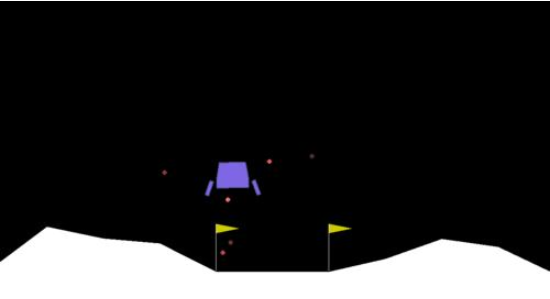## A2C 算法的基本结构

A2C 是 openAI 在实现baseline过程中提出的，是一种结合了 Value-based (比如 Q learning) 和 Policy-based (比如 Policy Gradients) 的强化学习算法。

Actor 目的是学习策略函数π(θ)以得到尽量高的回报。 Critic 目的是对当前策略的值函数进行估计，来评价。

Policy Gradient 算法的整个过程可以看作先通过策略π(θ)让 agent 与环境进行互动，计算每一步所能得到的奖励，并以此得到一局游戏的奖励作为累积奖励 G，然后通过调整策略π，使得 G 最大化。所以使用了梯度提升的方法来更新网络参数θ，利用更新后的策略再采集数据，再更新，如此循环，达到优化策略的目的。

• Actor Critic

agent 在于环境互动过程中产生的 G 值本身是一个随机变量，可以通过 Q 函数去估计 G 的期望值，来增加稳定性。即 Actor-Critic 算法在 PG 策略的更新过程中使用 Q 函数来代替了 G，同时构建了 Critic 网络来计算 Q 函数，此时 Actor 相关参数的梯度为：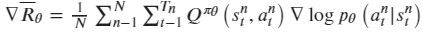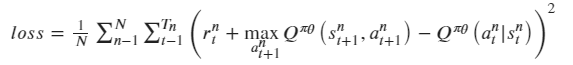• A2C 算法

A2C 在 AC 算法的基础上使用状态价值函数给 Q 值增加了基线 V，使反馈可以为正或者为负，因此 Actor 的策略梯变为：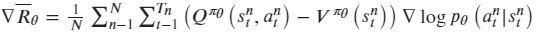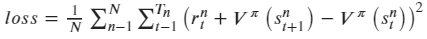## LunarLander-v2 游戏环境简介

LunarLander-v2，是基于 gym 和 box2d 提供的游戏环境。游戏任务为玩家通过操作登月器的喷气主引擎和副引擎来控制登月器降落。

gym:开源强化学习 python 库，提供了算法和环境交互的标准 API，以及符合该 API 的标准环境集。

box2d:gym 提供的一种环境集合

## 注意事项

1. 本案例运行环境为 TensorFlow-1.13.1，且需使用 GPU 运行，请查看《ModelAtrs JupyterLab 硬件规格使用指南》了解切换硬件规格的方法；

2. 如果您是第一次使用 JupyterLab，请查看《ModelAtrs JupyterLab使用指导》了解使用方法；

3. 如果您在使用 JupyterLab 过程中碰到报错，请参考《ModelAtrs JupyterLab常见问题解决办法》尝试解决问题。

## 实验步骤

### 1. 程序初始化

!pip install gym!conda install swig -y!pip install box2d-py!pip install gym[box2d]

import osimport gymimport numpy as npimport tensorflow as tfimport pandas as pd

### 2. 参数设置

MAX_EPISODE = 100               # 游戏最大局数DISPLAY_REWARD_THRESHOLD = 100  # 开启可视化的reward阈值SAVE_REWARD_THRESHOLD = 100     # 保存模型的reward阈值MAX_EP_STEPS = 2000             # 每局最大步长TEST_EPISODE = 10               # 测试局RENDER = False                  # 是否启用可视化（耗时）GAMMA = 0.9                     # TD error中reward衰减系数RUNNING_REWARD_DECAY=0.95       # running reward 衰减系数LR_A = 0.001                    # Actor网络的学习率LR_C = 0.01                     # Critic网络学习率NUM_UNITS = 20                  # FC层神经元个数SEED = 1                        # 种子数，减小随机性SAVE_EPISODES = 20              # 保存模型的局数model_dir = './models'          # 模型保存路径

### 3. 游戏环境创建

def create_env():    env = gym.make('LunarLander-v2')    # 减少随机性    env.seed(SEED)    env = env.unwrapped    num_features = env.observation_space.shape    num_actions = env.action_space.n    return env, num_features, num_actions

### 4. Actor-Critic 网络构建

class Actor:    """    Actor网络    Parameters    ----------    sess : tensorflow.Session()    n_features : int        特征维度    n_actions : int        动作空间大小    lr : float        学习率大小    """    def __init__(self, sess, n_features, n_actions, lr=0.001):        self.sess = sess        # 状态空间        self.s = tf.placeholder(tf.float32, [1, n_features], "state")        # 动作空间        self.a = tf.placeholder(tf.int32, None, "action")        # TD_error        self.td_error = tf.placeholder(tf.float32, None, "td_error")        # actor网络为两层全连接层，输出为动作概率        with tf.variable_scope('Actor'):            l1 = tf.layers.dense(                inputs=self.s,                units=NUM_UNITS,                activation=tf.nn.relu,                kernel_initializer=tf.random_normal_initializer(0., .1),                bias_initializer=tf.constant_initializer(0.1),                name='l1'            )            self.acts_prob = tf.layers.dense(                inputs=l1,                units=n_actions,                activation=tf.nn.softmax,                kernel_initializer=tf.random_normal_initializer(0., .1),                bias_initializer=tf.constant_initializer(0.1),                name='acts_prob'            )        with tf.variable_scope('exp_v'):            log_prob = tf.log(self.acts_prob[0, self.a])            # 损失函数            self.exp_v = tf.reduce_mean(log_prob * self.td_error)        with tf.variable_scope('train'):             # minimize(-exp_v) = maximize(exp_v)            self.train_op = tf.train.AdamOptimizer(lr).minimize(-self.exp_v)    def learn(self, s, a, td):        s = s[np.newaxis, :]        feed_dict = {self.s: s, self.a: a, self.td_error: td}        _, exp_v = self.sess.run([self.train_op, self.exp_v], feed_dict)        return exp_v    # 生成动作    def choose_action(self, s):        s = s[np.newaxis, :]        probs = self.sess.run(self.acts_prob, {self.s: s})          return np.random.choice(np.arange(probs.shape), p=probs.ravel())class Critic:    """    Critic网络    Parameters    ----------    sess : tensorflow.Session()    n_features : int        特征维度    lr : float        学习率大小    """    def __init__(self, sess, n_features, lr=0.01):        self.sess = sess        # 状态空间        self.s = tf.placeholder(tf.float32, [1, n_features], "state")        # value值                self.v_ = tf.placeholder(tf.float32, [1, 1], "v_next")        # 奖励                   self.r = tf.placeholder(tf.float32, None, 'r')        # critic网络为两层全连接层，输出为value值        with tf.variable_scope('Critic'):            l1 = tf.layers.dense(                inputs=self.s,                # number of hidden units                units=NUM_UNITS,                activation=tf.nn.relu,                 kernel_initializer=tf.random_normal_initializer(0., .1),                  bias_initializer=tf.constant_initializer(0.1),                  name='l1'            )            self.v = tf.layers.dense(                inputs=l1,                 # output units                units=1,                activation=None,                kernel_initializer=tf.random_normal_initializer(0., .1),                  bias_initializer=tf.constant_initializer(0.1),                 name='V'            )        with tf.variable_scope('squared_TD_error'):            self.td_error = self.r + GAMMA * self.v_ - self.v            # TD_error = (r+gamma*V_next) - V_eval            self.loss = tf.square(self.td_error)        with tf.variable_scope('train'):            self.train_op = tf.train.AdamOptimizer(lr).minimize(self.loss)    def learn(self, s, r, s_):        s, s_ = s[np.newaxis, :], s_[np.newaxis, :]        v_ = self.sess.run(self.v, {self.s: s_})        td_error, _ = self.sess.run([self.td_error, self.train_op],                                    {self.s: s, self.v_: v_, self.r: r})        return td_error

### 5. 创建训练函数

def model_train():    env, num_features, num_actions = create_env()    render = RENDER    sess = tf.Session()    actor = Actor(sess, n_features=num_features, n_actions=num_actions, lr=LR_A)    critic = Critic(sess, n_features=num_features, lr=LR_C)    sess.run(tf.global_variables_initializer())    saver = tf.train.Saver()    for i_episode in range(MAX_EPISODE+1):        cur_state = env.reset()        cur_step = 0        track_r = []        while True:            # notebook暂不支持该游戏的可视化            # if RENDER:            # env.render()            action = actor.choose_action(cur_state)            next_state, reward, done, info = env.step(action)            track_r.append(reward)            # gradient = grad[reward + gamma * V(next_state) - V(cur_state)]            td_error = critic.learn(cur_state, reward,                                    next_state)            # true_gradient = grad[logPi(cur_state,action) * td_error]            actor.learn(cur_state, action, td_error)              cur_state = next_state            cur_step += 1            if done or cur_step >= MAX_EP_STEPS:                ep_rs_sum = sum(track_r)                if 'running_reward' not in locals():                    running_reward = ep_rs_sum                else:                    running_reward = running_reward * RUNNING_REWARD_DECAY + ep_rs_sum * (1-RUNNING_REWARD_DECAY)                # 判断是否达到可视化阈值                # if running_reward > DISPLAY_REWARD_THRESHOLD:                #     render = True                print("episode:", i_episode, "  reward:", int(running_reward), "  steps:", cur_step)                break        if i_episode > 0 and i_episode % SAVE_EPISODES == 0:            if not os.path.exists(model_dir):                os.mkdir(model_dir)            ckpt_path = os.path.join(model_dir, '{}_model.ckpt'.format(i_episode))            saver.save(sess, ckpt_path)

### 6. 开始训练

print('MAX_EPISODE:', MAX_EPISODE)model_train()# reset graphtf.reset_default_graph()

### 7.使用模型推理

def model_test():    env, num_features, num_actions = create_env()    sess = tf.Session()    actor = Actor(sess, n_features=num_features, n_actions=num_actions, lr=LR_A)    sess.run(tf.global_variables_initializer())    saver = tf.train.Saver()    saver.restore(sess, tf.train.latest_checkpoint(model_dir))    for i_episode in range(TEST_EPISODE):        cur_state = env.reset()        cur_step = 0        track_r = []        while True:            # 可视化            # env.render()            action = actor.choose_action(cur_state)            next_state, reward, done, info = env.step(action)            track_r.append(reward)            cur_state = next_state            cur_step += 1            if done or cur_step >= MAX_EP_STEPS:                ep_rs_sum = sum(track_r)                print("episode:", i_episode, "  reward:", int(ep_rs_sum), "  steps:", cur_step)                break

model_test()episode: 0   reward: -31   steps: 196episode: 1   reward: -99   steps: 308episode: 2   reward: -273   steps: 533episode: 3   reward: -5   steps: 232episode: 4   reward: -178   steps: 353episode: 5   reward: -174   steps: 222episode: 6   reward: -309   steps: 377episode: 7   reward: 24   steps: 293episode: 8   reward: -121   steps: 423episode: 9   reward: -194   steps: 286

### 8.可视化效果## 评论